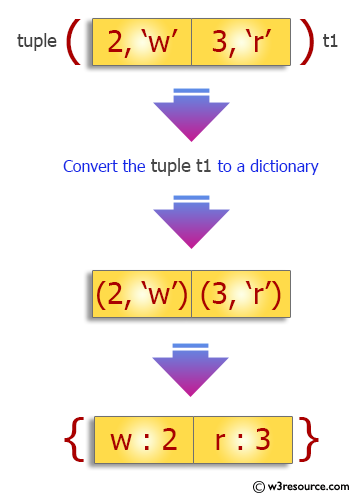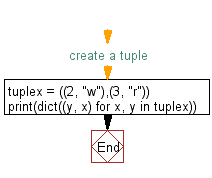﻿ Python: Convert a tuple to a dictionary - w3resource# Python Exercise: Convert a tuple to a dictionary

## Python tuple: Exercise-16 with Solution

Write a Python program to convert a tuple to a dictionary.

Sample Solution:-

Python Code:

``````#create a tuple
tuplex = ((2, "w"),(3, "r"))
print(dict((y, x) for x, y in tuplex))
```
```

Sample Output:

```{'w': 2, 'r': 3}
```

Pictorial Presentation:Flowchart:## Visualize Python code execution:

The following tool visualize what the computer is doing step-by-step as it executes the said program:

Python Code Editor:

Have another way to solve this solution? Contribute your code (and comments) through Disqus.

What is the difficulty level of this exercise?

Test your Programming skills with w3resource's quiz.

﻿

## Python: Tips of the Day

Decapitalizes the first letter of a string:

Example:

```def tips_decapitalize(s, upper_rest=False):
return s[:1].lower() + (s[1:].upper() if upper_rest else s[1:])
print(tips_decapitalize('PythonTips'))
print(tips_decapitalize('PythonTips', True))
```

Output:

```pythonTips
pYTHONTIPS
```